Register for Maths, Science, English, Reasoning Olympiad Exams Register here | Book Free Demo for Live Olympiad Classes here | Check Olympiad Exam Dates here | Buy Practice Papers for IMO, IOM, HEO, IOEL etc here | Login here to participate in all India free mock test on every Saturday

# Coordinate Geometry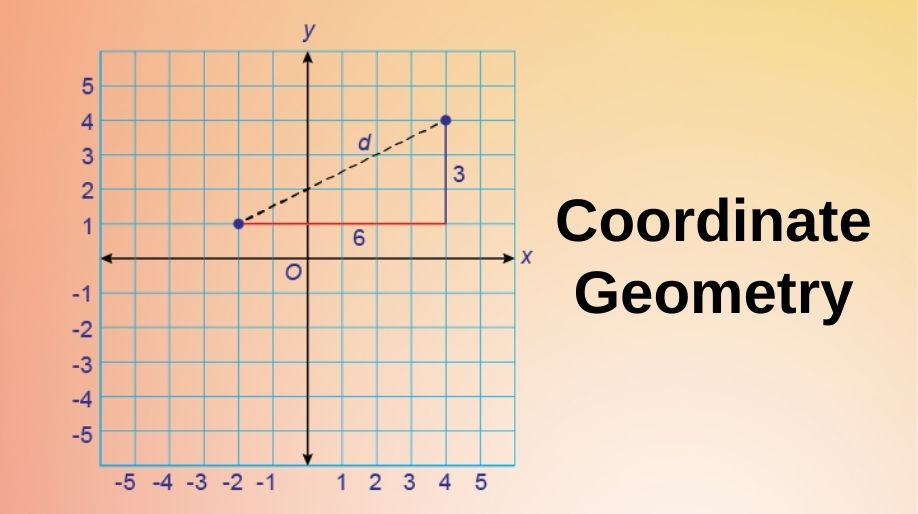In the field of mathematics every point, any figure, plane or anything which has physical significance has a specific position in space. To locate any point we require a proper position of the point and these positions are termed as coordinates. Here we will learn about the geometries of various figures, their representation through the coordinates.

Coordinate Geometry is the determination of the coordinates of any figure, plane or any point in space and application of the various geometries on these figures.

## Cartesian System

• In 1637 French Scientist René Déscartes solved the problem of describing the position of a point in a plane.
• Descartes invented the idea of placing two such lines perpendicular to each other on a plane and locating points on the plane by referring them to these lines.
• His method was a development of the older idea of latitude and longitude.
• In honor of Déscartes, the system used for describing the position of a point in a plane is also known as the Cartesian system.
• The two perpendicular lines orientation was a major problem and that’s why we have taken a standard measurement that is the two perpendicular lines will be the horizontal and vertical axis.
• Combine both the lines in such a way that the two lines cross each other at their zeroes which we call as Origin.
• The horizontal line X’X is called the x-axis and the vertical line Y’Y is called the y-axis. The point where X’X and Y’Y cross is called the origin and is denoted by O.
• Since the positive numbers lie on the directions OX and OY, OX and OY are called the positive directions of the x-axis and the y-axis, respectively.
• Similarly, OX and OY are called the negative directions of the x-axis and the y-axis, respectively.
• The following figure shows the Cartesian system.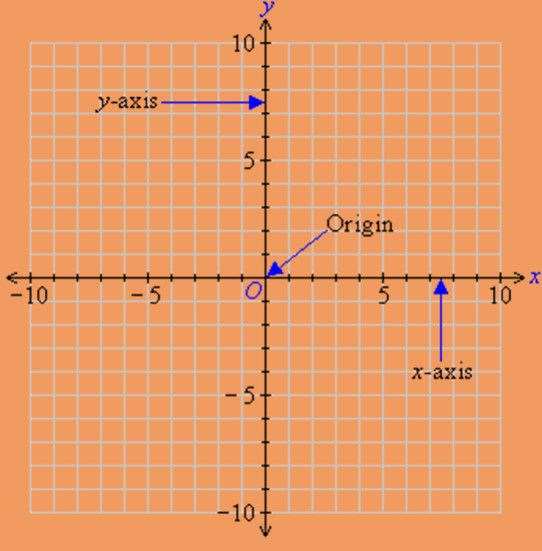### Cartesian Plane and Terminology Involved

The Cartesian plane is a rectangular coordinate system that associates each point in the plane with a pair of numbers.

The two perpendicular lines are called as the coordinate axis. The horizontal line is known as the X-axis and the vertical line is known as the Y-axis.

The coordinate axis divides the Cartesian plane into four parts which are called as the quadrants.

The point of intersection of the coordinate axis is called as the origin.

• As we can see that the figure is divided into four parts by the two perpendicular lines.
• The figure below shows the names of all the four parts: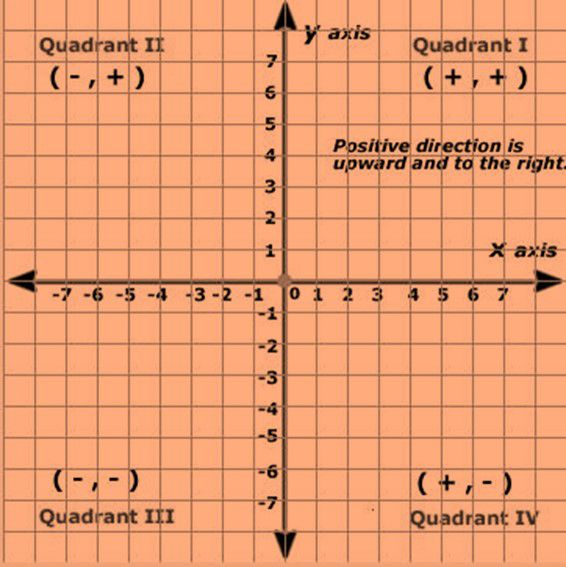• Each of the part is called as a quadrant.

Let us now learn how do we write the coordinates of a point in the Cartesian plane.

• The position of any point or figure is expressed in the Cartesian with the help of certain measurements which are called as coordinates.
• Coordinates are basically the distance of the point from the x and y-axis respectively.
• The x - coordinate of a point is its perpendicular distance from the y-axis measured along the x-axis which is positive along the positive direction of the x-axis and negative along the negative direction of the x –
• The y - coordinate of a point is its perpendicular distance from the x-axis measured along the y-axis (positive along the positive direction of the y-axis and negative along the negative direction of the y-axis).
• The x coordinate is also called as abscissa.
• The y coordinate is also called as the ordinate.
• While writing or expressing the coordinates of a point in the coordinate plane, the x – coordinate comes first, and then the y - coordinate. We place the coordinates in brackets.
• In the first quadrant, both of the coordinates of the point are positive.
• In the second quadrant, the x coordinate is negative while y coordinate is positive.
• In the third quadrant, the x coordinate and y coordinate both are negative.
• In the fourth quadrant, the x coordinate is positive and the y coordinate is negative.

Coordinate System

Here we are going to learn about how we are going to represent a point if we have been given the coordinates:

Example 1: Locate the point P (3,5) on the Cartesian plane.

Solution: Here on observing the coordinates we can conclude that the abscissa is 3 and the ordinate is 5.

It means that the distance from the Y-axis is 3 and the distance from the X-axis is 5.

Hence the representation will be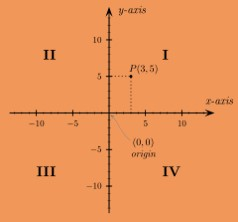### Practice Questions

Q1) Locate the following points in the Cartesian plane.

1. A(1,2)
2. B(8,-5)
3. C(-7,2)
4. D(-4,-4)

Q2) From the following figure observe the points and find their coordinates.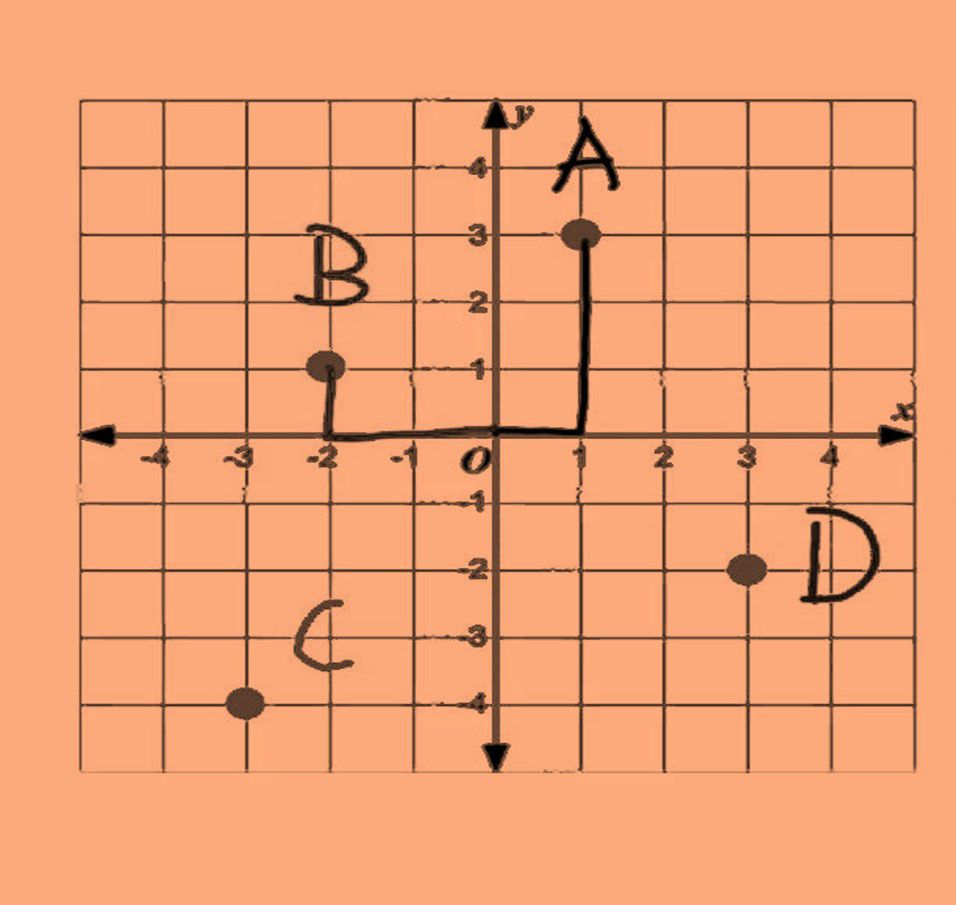## Recap

• Coordinate Geometry is the determination of the coordinates of any figure, plane or any point in space and application of the various geometries on these figures.
• Descartes invented the idea of placing two such lines perpendicular to each other on a plane and locating points on the plane by referring them to these lines.
• The coordinate axis divides the Cartesian plane into four parts which are called as the quadrants.
• The Cartesian plane is a rectangular coordinate system that associates each point in the plane with a pair of numbers.
• While writing or expressing the coordinates of a point in the coordinate plane, the x – coordinate comes first, and then the y - coordinate. We place the coordinates in brackets.
• The coordinates of the origin are (0, 0).

## Quiz for Coordinate Geometry

 Q.1 A (-2, 4) and B (-4, 2) are reflected in the y axis. If A' and B' are the images of A and B respectively, the co-ordinates of A' and B' are a) (-2, -4), (-4, -2) b) (2, 4), (4, 2) c) (-2, 4), (-4, 2) d) (2, -4), (4, -2)
 Q.2 P (2, -4) is reflected about the y axis to get the image Q. Q is reflected about the x axis to get the image R. Name the figure PQR. a) Equilateral triangle b) Right Angled Scalene Triangle c) Right Angled Isosceles Triangle d) none of the above
 Q.3 P (5, 3) was reflected in the origin to get P'. M is the foot of perpendicular from P to x axis. N is the foot of perpendicular from P' to x axis. The figure PMP'N is a) Parallelogram b) Kite c) Rhombus d) Rectangle
 Q.4 P (3, 4) is reflected to P' in the x axis. And O' is the image of origin when reflected in the line PP'. Coordinate of O' is a) (5, 0) b) (6, 0) c) (-6, 0) d) (-5, 0)
 Q.5 The triangle OAB is reflected in the origin to triangle OA'B'. A' is (-3, -4) and B' is (0, -5). Answer the following question: Coordinates of A and B are a) (3, 4) and (0, 5) b) (3, -4) and (0, 5) c) (-3, 4) and (5, 0) d) (3, 4) and (5, 0)
 Q.6 The triangle OAB is reflected in the origin to triangle OA'B'. A' is (-3, -4) and B' is (0, -5). Answer the following question: Find coordinate of A", the reflection of A in the origin followed by reflection in the y axis. a) (3, 4) b) (-3, -4) c) (3, -4) d) (-3, 4)
 Q.7 The distance between (7, 3) and another point on the x axis whose abscissa is 11 is a) 5 b) 5√3 c) 5√2 d) 5/√2
 Q.8 The distance between A (1, 3) and B (x, 7) is 5. the possible values of x are a) 5 or -3 b) 4 or -2 c) 3 or -1 d) 2 or 0
 Q.9 The points (1, 1), (-1, -1) and (-√3, √3) form a) scalene triangle b) isosceles triangle c) right angled triangle d) equilateral triangle
 Q.10 If the points (0, 3), (-2, a) and (-1, 4) are the vertices of a right angled triangle at the first point, the value of a is a) 1 b) 2 c) 3 d) 4